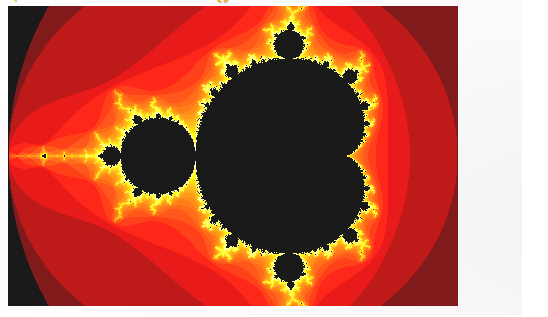Manuals/calci/MANDELBROT

Description

• This function shows the figure of the Mandelbrot.
• Fractals are infinitely complex patterns that are self-similar across different scales.
• This property is called self-similarity.
• Fractals form a never ending pattern, created by repeating a simple process over and over, in an ongoing feedback loop.Mandelbrot Set is the set of points in the complex plane with the sequencewhere the result does not approach infinity.
• The Julia Set is closely related to Mandelbrot Set.
• The Mandelbrot Set is obtained from the quadratic recurrence equation, (with=0), where points c in the complex plane for which the computed value ofdoes not tend to infinity.

Examples

1. MANDELBROT()Mandel brot